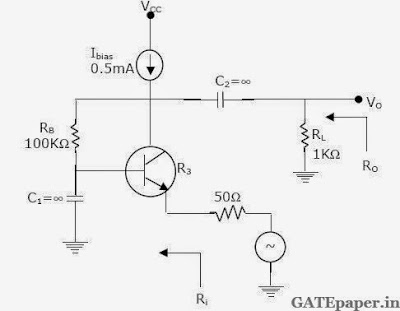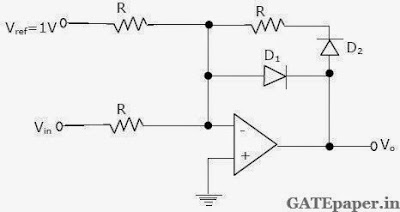### GATE 1999 ECE Video Solutions on Analog Circuits (Analog Electronics)

1. The first pole encountered in the frequency response of a compensated OP-AMP is approximately at
a. 5 Hz
b. 10 kHz
c. 1 MHz
d. 100 MHz

2. Negative feedback in an amplifier
a. Reduces gain
b. Increases frequency and phase distortions
c. Reduces bandwidth
d. Increases noise

3. Crossover distortion behavior is characteristic of
a. Class A output stage
b. Class B output stage
c. Class AB output stage
d. Common base output stage

4. An NPN transistor (with C = 0.3 pF) has a unity gain cutoff frequency fτ of 400 MHz at a dc bias current IC = 1 mA. The value of its Cµ (in pF) is approximately (VT = 26 mV)
a. 15
b. 30
c. 50
d. 96

5. An amplifier has an Open loop gain of 100, an input impedance of 1 kΩ and an output impedance of 100 Ω. A feedback network with a feedback factor of 0.99 is connected to the amplifier in a voltage series feedback mode. The new input and output impedances, respectively are
a. 10 Ω and 1 Ω
b. 10 Ω and 10 kΩ
c. 100 kΩ and 1 Ω
d. 100 kΩ and 1 kΩ

6. An amplifier is assumed to have a single pole high frequency transfer function. The rise time of its output response to a step function input is 35 nsec. The upper -3 dB frequency (in MHz) for the amplifier to a sinusoidal input is approximately at
a. 4.55
b. 10
c. 20
d. 28.6

7. A bipolar junction transistor amplifier is shown below. Assume that the current source Ibias is ideal, and the transistor has very large β, rb = 0 and r0 -> ∞.Determine the ac small signal mid band voltage gain (Vo / Vs), input resistance (Ri) and output resistance (Ro) of the circuit. Assume VT = 26 mV.

8. A JFET having µ = 50 and rd = 10 kΩ is used in a common source configuration as shown. The JFET capaciances are Cgs = 5 pF, Cgd = 2 pF and Cds = 2 pF.Determine the ac small signal mid band voltage gain (Vo/Vs) and the upper 3 dB frequency of the circuit.

9. Neatly sketch and label the DC transfer characteristic (Vo verses Vin) of the circuit shown, as Vin varies from – 2 volts to + 2 volts.Assume ideal operational amplifier and the diodes have a forward voltage of 0.6 volts and zero incremental resistance.

10. A transistor LC oscillator circuit is shown below.Assume that the transistor has very high value of β(so that you may neglect rb). Derive an equation governing the circuit operation, and find the frequency of oscillation. Also state the gain condition required for oscillation to start.